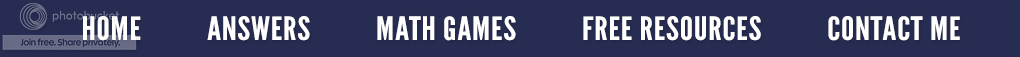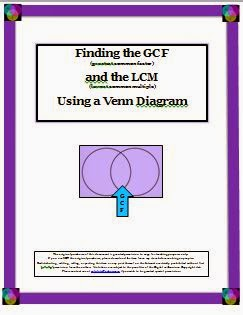### Finding the Greatest Common Factor and Least Common Multiple

The most common method to find the greatest common factor (GCF) is to list all of the factors of each number, then list the common factors and choose the largest one.  Example: Find the GCF of 36 and 54.

1) The factors of 36 are: 1, 2, 3, 4, 6, 9, 12, 18, and 36.

2) The factors of 54 are: 1, 2, 3, 6, 9, 18, 27, and 54.

Therefore, the common factor(s) of 36 and 54 are: 1, 2, 3, 6, 9, 18.  Although the numbers in bold are all common factors of 36 and 54, 18 is the greatest common factor.

To find the lowest common multiple (LCM), students are asked to list all of the factors of the given numbers. Let's say the numbers are 9 and 12.

1) The multiples of 9 are: 9, 18, 27, 36, 45, 54.

2) The multiples of 12 are: 12, 24,  36, 48, 60.

As seen above, the least common multiple for these two numbers is 36.  I have seen this done on a large letter M as illustrated below.

We often instruct our students to first list the prime factors, then multiply the common prime factors to find the GCF. Often times, if just this rule is given, students become lost in the process. Utilizing a visual can achieve an understanding of any concept better than just a rule. A two circle Venn Diagram is such a visual and will allow students to follow the process as well as to understand the connection between each step. For example: Let’s suppose we have the numbers 18 and 12.

1) Using factor trees, the students list all the factors of each number.

2) Now they place all the common factors in the intersection of the two circles. In this case, it would be the numbers 2 and 3.

3) Now the students place the remaining factors in the correct big circle(s).

4) That leaves the 18 with a 3 all by itself in the big circle. The 12 has just a 2 in the big circle.

5) The intersection is the GCF; therefore, multiply 2 × 3 to find the GCF of  6.

6) To find the LCM, multiply the number(s) in the first big circle by the GCF (numbers in the intersection) times the number (s) in the second big circle.

3 × GCF × 2 = 3 × 6 × 2 = 36. The LCM is 36.

This is an effective method to use when teaching how to reduce fractions,Finding GCF and LCM
I have turned this method into a resource for Teachers Pay Teachers. It is 16 pages and begins with defining the words factor, greatest common factor and least common multiple. What a factor tree is and how to construct and use a Venn Diagram as a graphic organizer is shown. Step-by-step examples are given as well as student practice pages. How to use a three circle Venn Diagram when given three different numbers is explained. Two pages of blank pages Venn Diagrams are included for classroom practice. To learn more, just click on the title under the resource cover on your right.

Next week's post - Finding the LCM and GCF for a set of algebraic terms.

### Randomly Grouping Students

My college students are like charter members of a church. They claim their seat on the first day of class, and from then on, no one else better take it! Since we journal every day, often in groups, the same people were sharing with the same people day after day. This meant the students were not getting to know each other; they were unaware of how others were problem solving, and they were way too comfortable in their group. Things had to change!

So-o-o I asked my students to make name tents by folding a large file card in half. (I know it sounds elementary, but it does help this "old" teacher to quickly learn who is who). On the outside, they printed their name and on the inside, I placed a variety of stickers.  (My students didn't seem to mind.) Based on the sticker I called, the students would group by pairs, threes, fours or groups of eight.  Now, the students would divide up into groups based on something other than their preference.

On Monday, we grouped by 2's according to the color of the dog and cat stickers. Right away I noticed that the dominance of the group had changed, and more dialogue was going on between the partners. We then regrouped to present problems using the order of operations, only this time I used the stars to make groups of 3's. (I used this same idea when I taught math in middle school and high school only the stickers were put directly onto the students' journals which always stayed in the room with me.)

I also used this strategy when I taught elementary (back in Noah's Day, after the flood), but there was always one or two "sticker pickers" in my class which seemed, in some magical way, to remove the sticker from their desk. To alleviate this problem, I placed the stickers on the desks and then covered them with clear packing tape or contact paper which was not easily removed. If a student moved away, I simply gave the new student the vacant desk or grouped the remaining students according to a different number.

Want to give this a try this in your classroom? Just purchase a variety of stickers. Then decide on the size of the groups you want such as 2's, 3's, 4's, 5's etc. and get to work!